# Contour plot in R

## The `contour` function

Given a matrix, and optionally the grid of X-axis and Y-axis values, it is possible to create a contour plot in R with the `contour` function.

``````# Data
x <- -10:10
y <- -10:10
z <- sqrt(outer(x ^ 2, y ^ 2, "+"))

contour(x, y, z)

# You can also type, the following
# but the axes will be between 0 and 1
contour(z)``````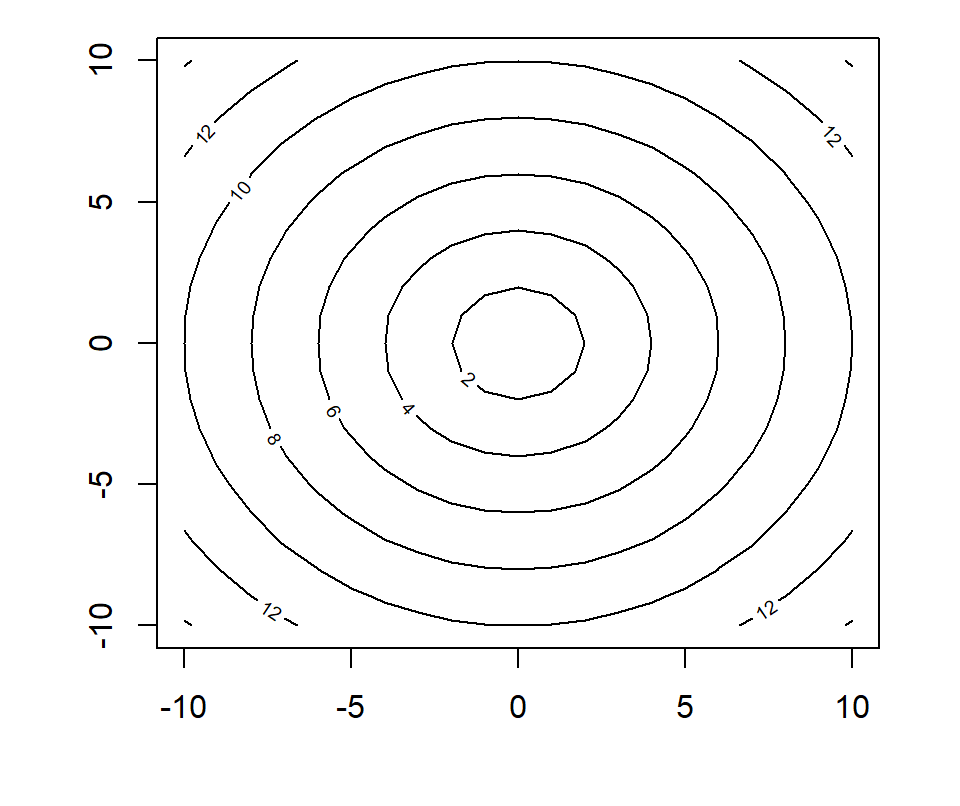Number of levels

The `nlevels` argument controls the number of contour levels to be drawn. The default value is 10.

``````# Data
x <- -10:10
y <- -10:10
z <- sqrt(outer(x ^ 2, y ^ 2, "+"))

contour(x, y, z,
nlevels = 20) ``````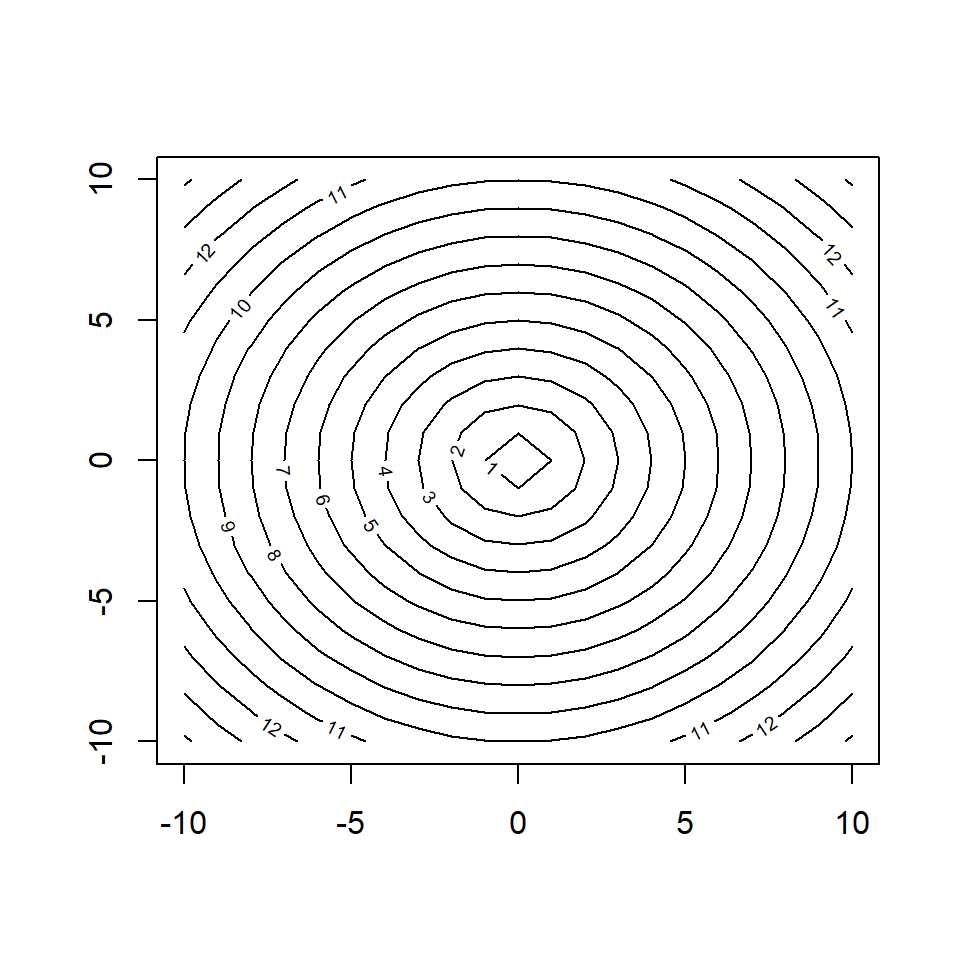Contour lines

Note that you can change the line width and line style of the contours with `lwd` and `lty`, respectively, as well as the size for contour labels with `labcex`, change the labels with `labels` or remove them setting `drawlabels = FALSE`.

``````# Data
x <- -10:10
y <- -10:10
z <- sqrt(outer(x ^ 2, y ^ 2, "+"))

contour(x, y, z,
labcex = 1.2, labels = 1:10,
lwd = 2, lty = 1) ``````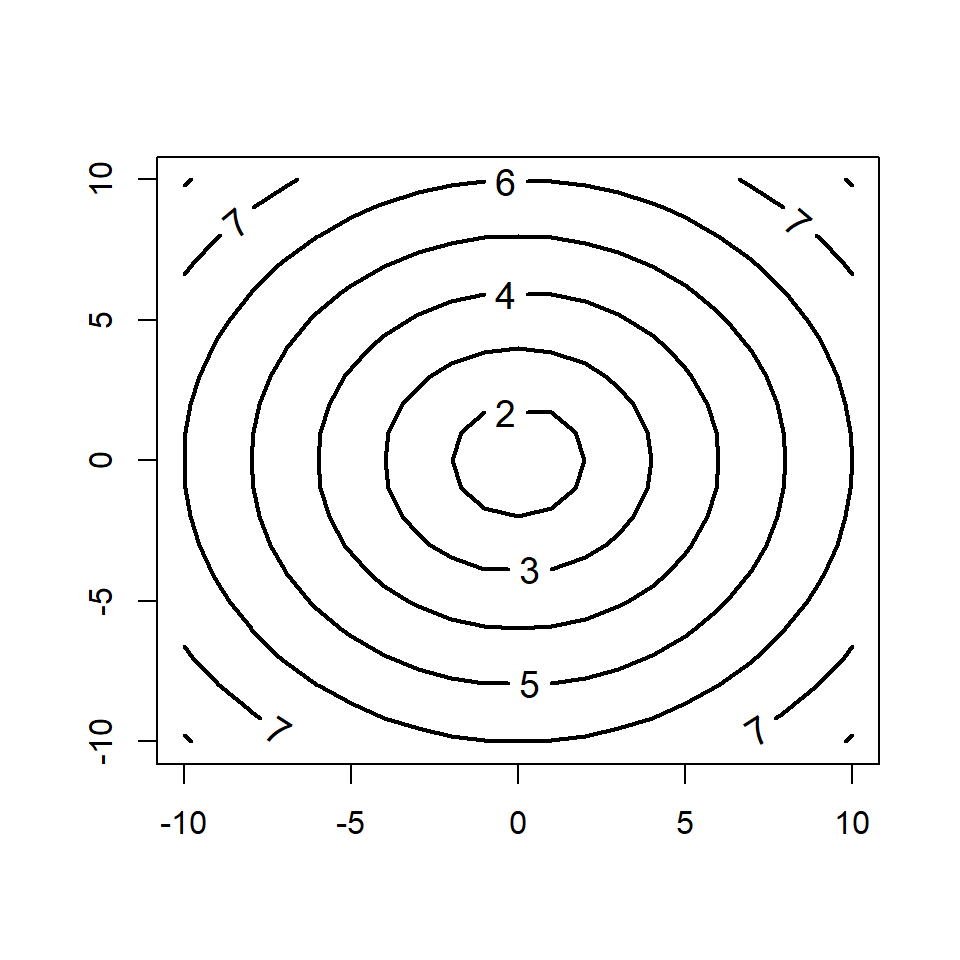Color palette

You can change the color of the contour lines with `col`, passing a color or a color palette, such in the example below, which draws contours for the `volcano` data set.

``````# Color palette
cols <- hcl.colors(10, "YlOrRd")

contour(volcano,
col = cols)``````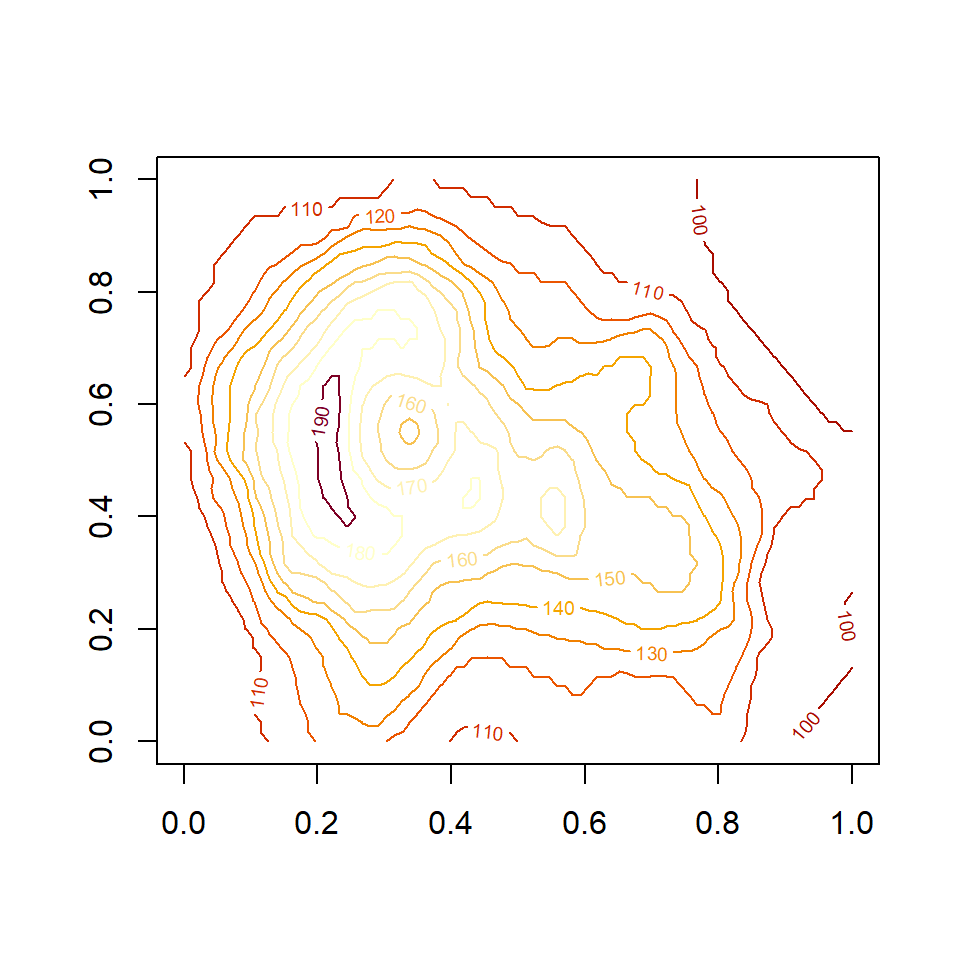Overlay contour over a scatter plot

If you want to overlay contour lines over a scatter plot you can use the `kde2d` function from `MASS` to calculate the kernel density estimate of the variables as shown in the example below. Recall to set `add = TRUE` inside `contour`.

``````# install.packages("MASS")
library(MASS)

# Data
x <- rnorm(500)
y <- rnorm(500)
z <- kde2d(x, y, n = 50)

plot(x, y, pch = 19)
contour(z, lwd = 2 add = TRUE,
col = hcl.colors(10, "Spectral"))``````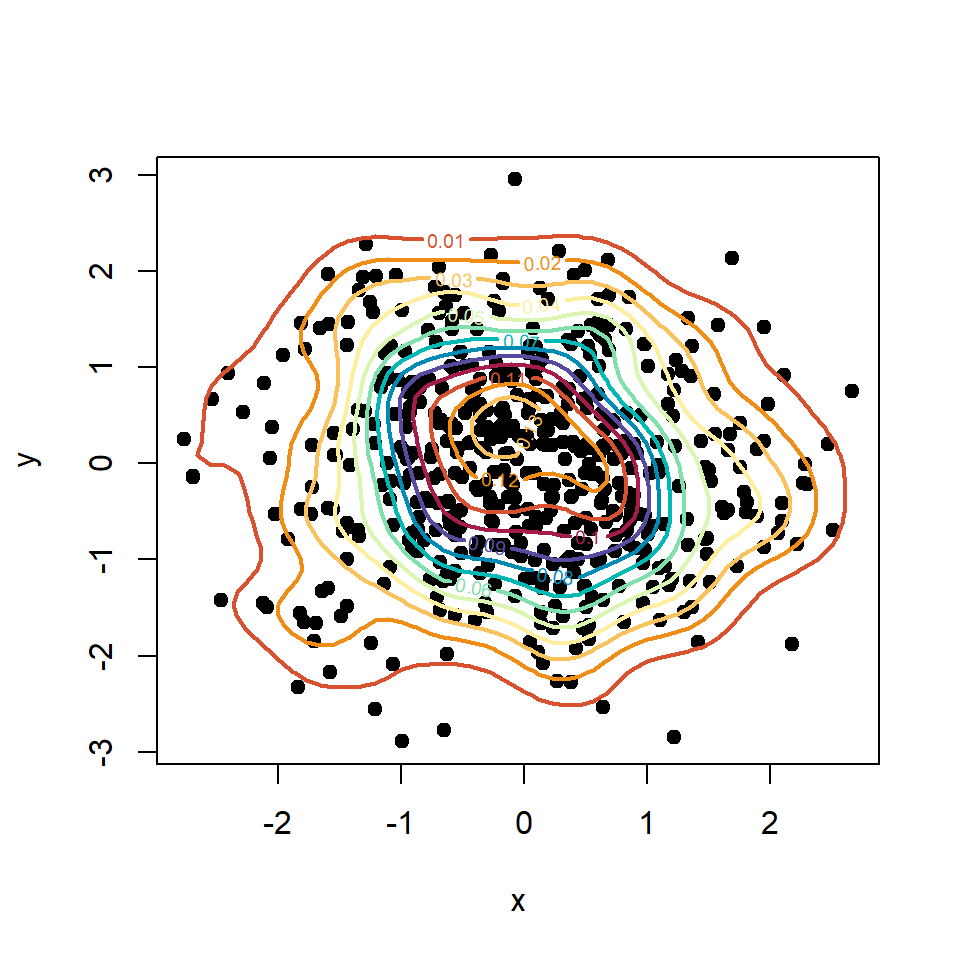## The `filled.contour` function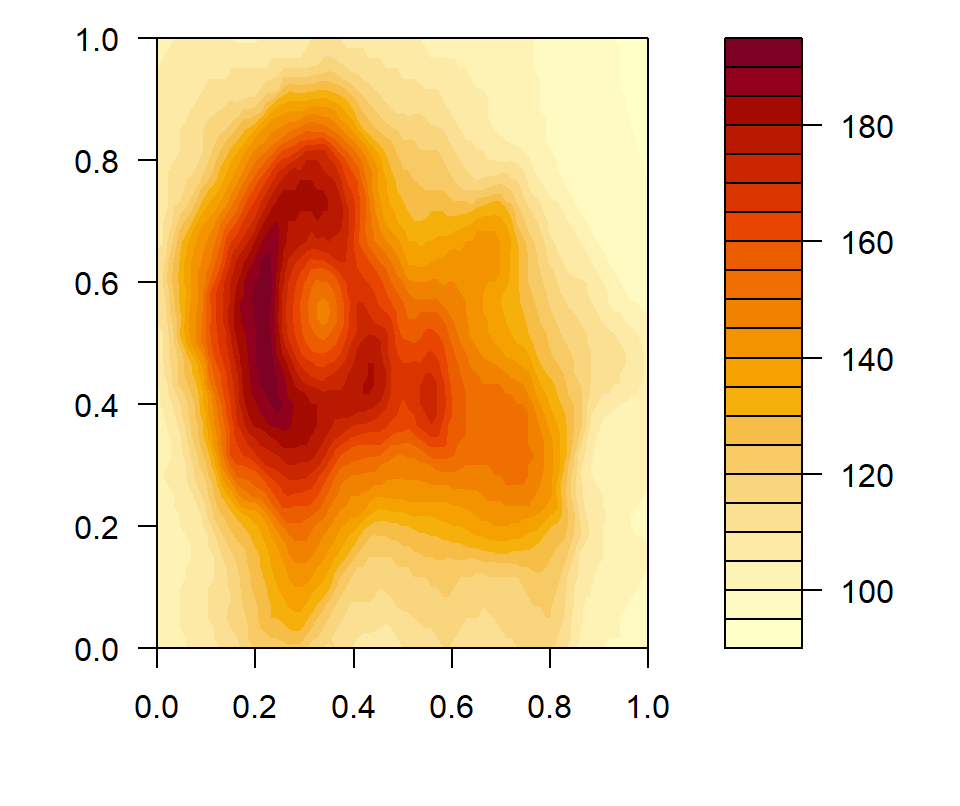A similar function to `contour` is `filled.contour`, which fills the areas between the contour lines. The function behaves almost the same as the function from the previous section.

``filled.contour(volcano)``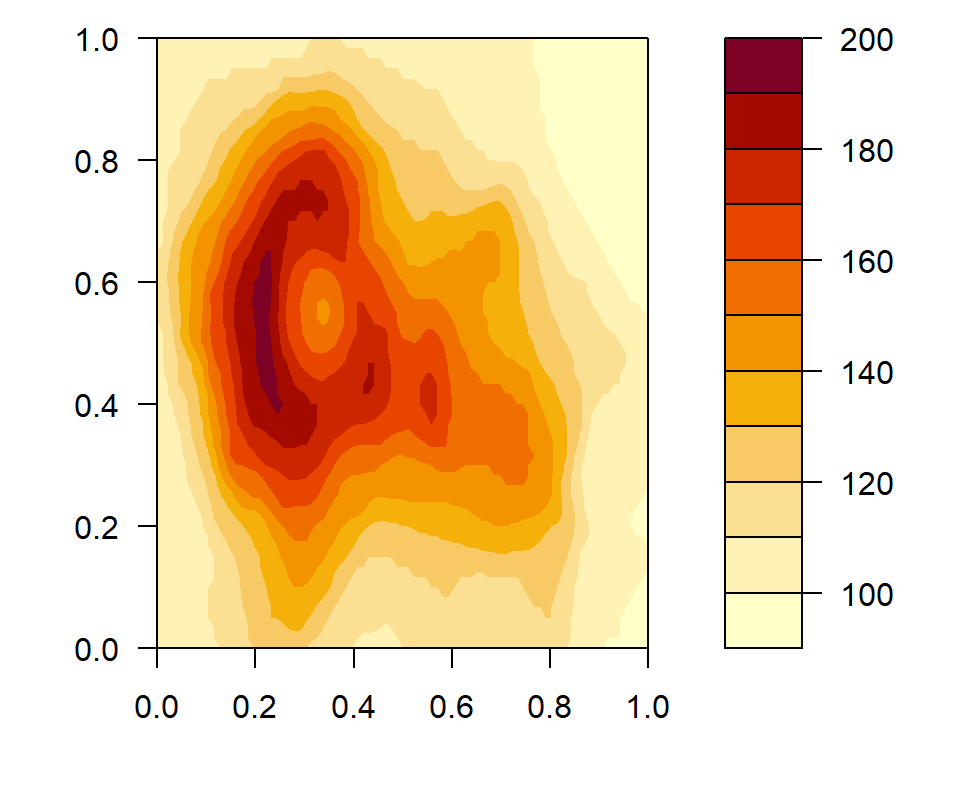Number of levels

In this function you can also specify the number of levels with `nlevels`.

``````filled.contour(volcano,
nlevels = 10)``````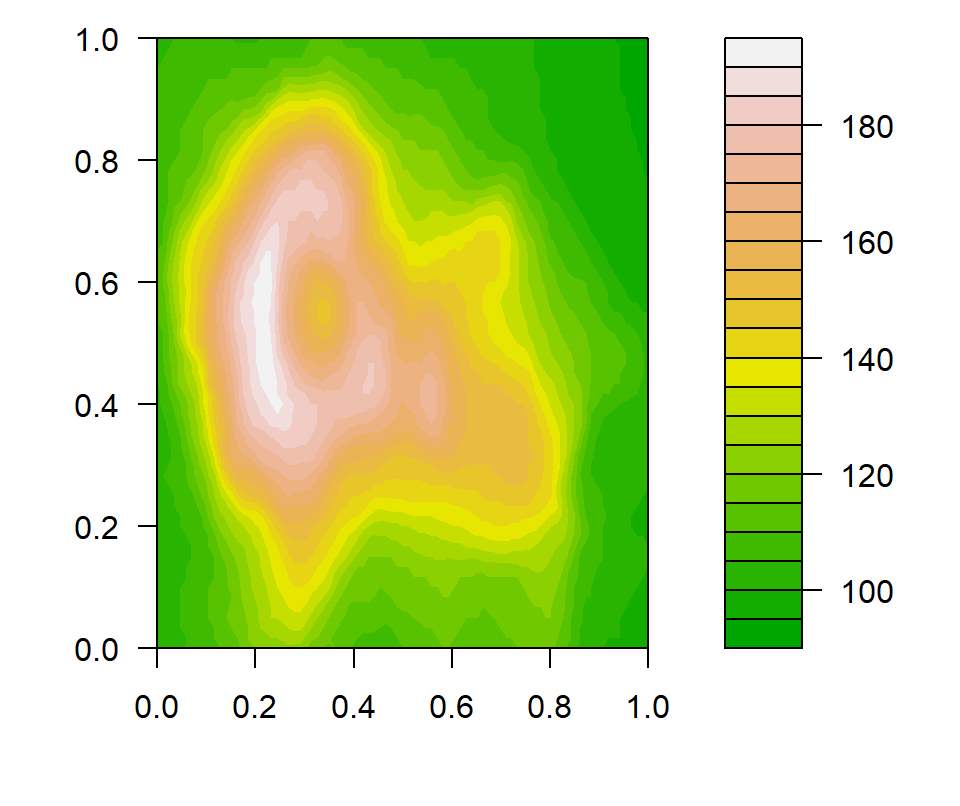Color palette

The color palette can be set with `color.palette` or specifying a vector of colors with `col`.

``````filled.contour(volcano,
color.palette = terrain.colors)``````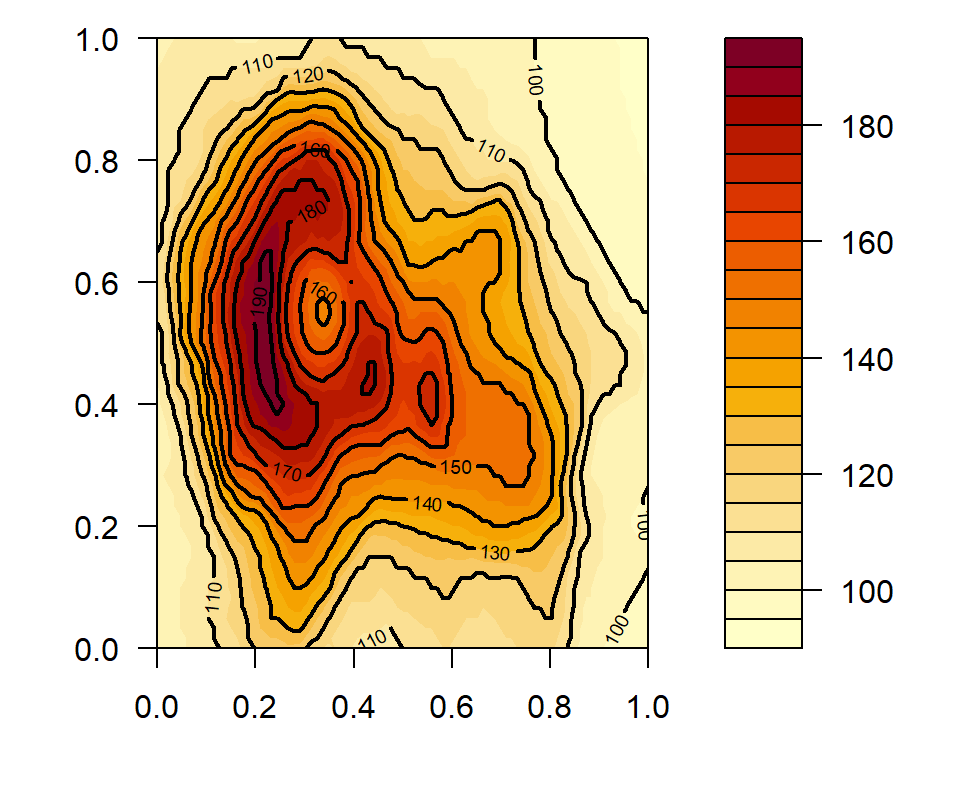Filled contour with lines

The `plot.axes` function can be used to add a contour over the filled contour plot, as shown in the following example.

``````filled.contour(volcano, plot.axes = {
axis(1)
axis(2)
contour(volcano, add = TRUE, lwd = 2)
}
)``````